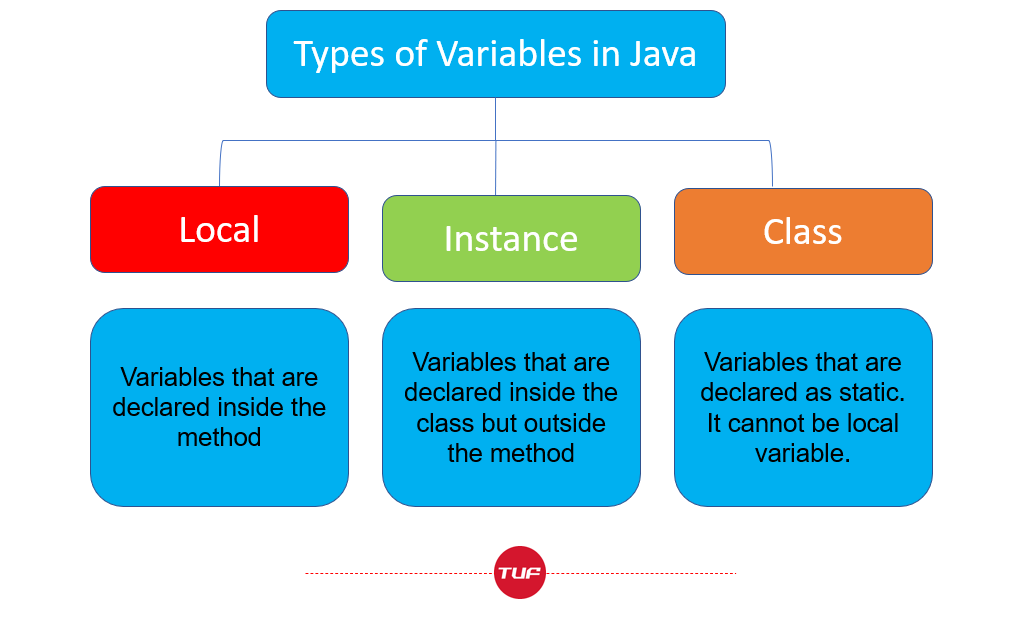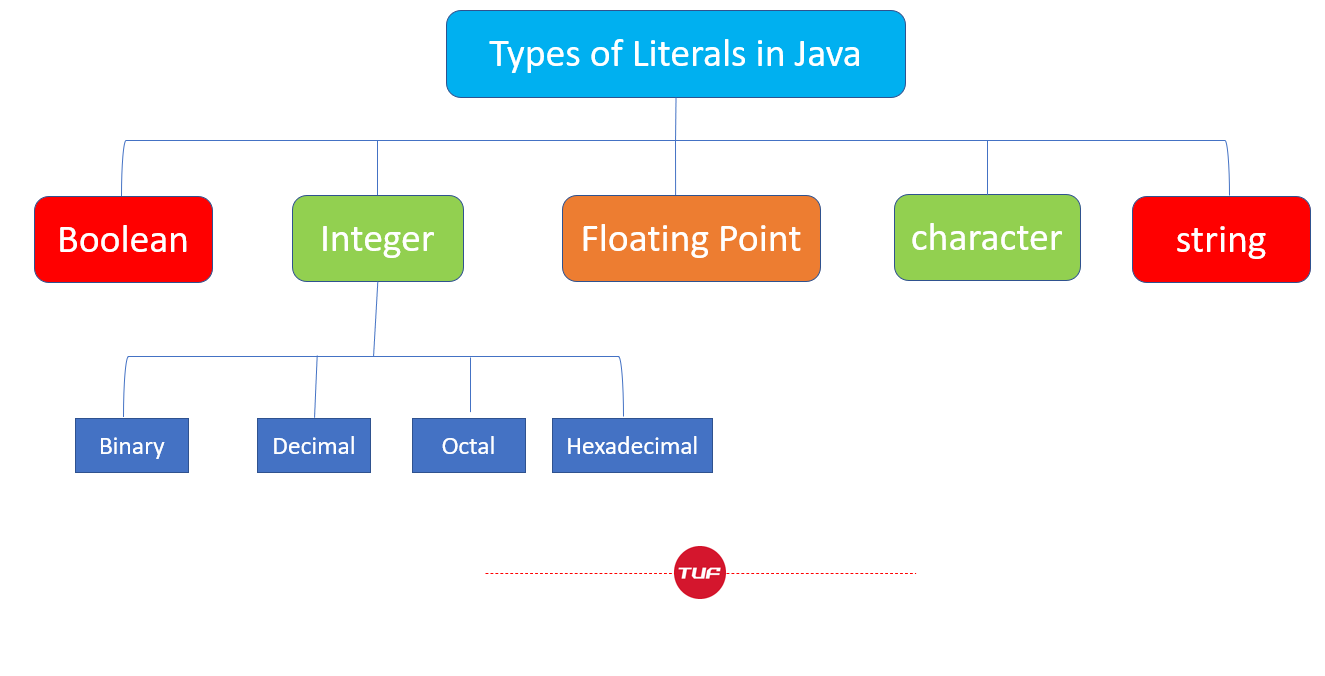# Java Variables and Literals

Java Variables

A variable is a memory location or storage area to hold the data.

Each variable should be given a unique Name (Identifier) to indicate the Storage Area.

Creating Variables in Java

` int tuf=150;`

This is the syntax for creating the variables:

`Datatype variableName = Value-assigned ; `

This means tuf is of datatype int and it is assigned value as 150.

Note – It is not necessary to create variables and assign values simultaneously.

``` int tuf;
tuf=50;
```

The above syntax is also correct.

Modifying Dataype of  Variables in Java

Java does not provide the facility for changing the data type of variables within the same scope.

So, it is not possible

Modifying Value of  Variables in Java

``` int tuf=1;
for(int i=0;i<5;i++)
{
tuf++; // Modifying value of tuf variable
}
tuf=2; // Modifying value of tuf variable
```

It is very easy to change the value of variables in Java.

Rules for Naming Variables in Java

Java Programming has its own set of rules and naming conventions for variables.

• Java is case sensitive Language. So, tuf and TUF are two different variables.
```int tuf=50;
int TUF=70;

System.out.println(tuf); // 50
System.out.println(TUF); // 70
```
• The naming convention which is common in almost all the languages – variables must start with either English Alphabets (a-z and A-Z), underscore (_), or a dollar sign (\$)
```// Valid Naming Conventions
int tuf;
int _tuf;
int \$tuf;
```
• Variables should not have whitespaces and they should not start with a number.
```// InValid Naming Conventions
int 1tuf;
int takeu forward;```
• Whenever assigning variable names, if we want to use a combination of words. Let’s say, we want to store the marks of Student
```Since, I cannot use variable as
int student marks; // since whitespaces are there

So, we create variable as studentmarks

But it is preferable to uncapitalize the first word and rest all subsequent words should be capitalized.

int studentMarks;
```
• For a single word variable, it is preferable to use all lowercase letters

Let’s say marks

```So instead of using MARKS , mARKS , it’s better to create it as marks
```
• It’s preferable to make variables with the name which defines their functionality . For Example,
```count - it describes we are counting something
Score - we are storing the score of a person
```

Types of  Variables in Java Programming Language### Java Literals

The constant or Fixed Value assigned to the variable is called Literal.

```int x=100; // here 100 is constant/literal

float val=4.7;
char ch='b';
```

Here 4.7 and ‘b’ are literals.

Here are the types of Literals in Java.• Boolean Literals

They are used to initialize boolean data types. They can store two values – either true or false.

```bool tuf=true;
bool takeuforward=false;```
• Integer Literals

They are numeric values without any exponential or fractional part. They are further classified into four categories

1. Binary ( base 2)
2. Decimal (base 10)
3. Octal (base 8 )
4. Hexadecimal (base 16)
```// Binary Number (Base 2)

int binary = 0b10100;

// Decimal Number (Base 10)

int decimal = 59;

// Octal Number (Base 8)

int Octal = 036;

// Hexadecimal Number (Base 16)

int Hexadecimal = 0x3E;
```

Here ,

0b -> Binary Number

0 -> Octal Number

0x -> Hexadecimal Number

NOTE – Integer literals are used to initialize variables of Integer types like int, long, short, and byte.

• Floating Point Literals

They represent values that are in exponential or fractional form.

```// Double Value
double tufdouble = 5.6;

// Float Value
float tuffloat = 5.6F;

// Exponential Value
float tufexpo= 2.7e3;```

Here , 2.7e3 represents -> 2.7 * 103

NOTE – Floating point literals are used to initialize float and double-type variables.

• Character Literals

They are Unicode characters that are placed inside the single quotes.

```// character literal

char ch='b';```

Here, b is character literal.

We can use escape sequences as character literals. For Example , \n (newline character) , \b (backspace) , \t (tab).

• String Literals

The sequence of characters placed inside the double quotes is known as a string literal.

`string tuf="Java is a good language";`

Java is a good language is a String literal.

Special thanks to Gurmeet Singh for contributing to this article on takeUforward. If you also wish to share your knowledge with the takeUforward fam, please check out this article